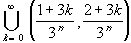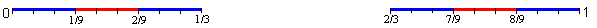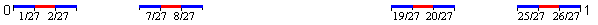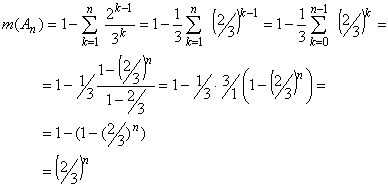#Interactive Real Analysis

Next | Previous | Glossary | Map

## 7.3. Measures

### Examples 7.3.10(c): Properties of Measure

Find the measure of the Cantor middle-third set (if it measurable).
Recall the definition of the Cantor set: let
A0 = [0, 1]
and define, for each n, the sets An recursively as
An = An-1 \Then the Cantor set is given as:
C =An
We have already shown that the Cantor set has "length" zero, but the concept of length does not really apply since the Cantor set is not an interval. Now we can redo our previous calculations, but under the mathematically correct name of measure.

First, the Cantor set is expressed as a combination of unions and intersections of intervals so that C is measurable. Let's compute the measure of the sets An:A0 = [0, 1] so that m(A0) = 1A1 = A0 - (1/3, 2/3) so that
m(A1) = m(A0) - m(1/3, 2/3) = 1 - 1/3A2 = A1 - (1/9, 2/9) - (7/9, 8/9) so that
m(A2) = m(A1) - m(1/9, 2/9) - m(7/9, 8/9) = 1 - 1/3 - 2*1/9A3 = A2 - (1/27, 2/27) - (7/27, 8/27) - (19/27, 20/25) - (25/27, 26/27) so that
m(A3) = 1 - 1/3 - 2*1/9 - 4*1/27
In general we havefor all n. Since CAn for all n we have that
m(C)(2/3)n
for all n. Therefore m(C) = 0.
Next | Previous | Glossary | Map